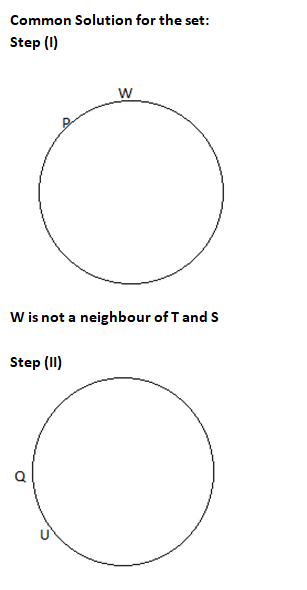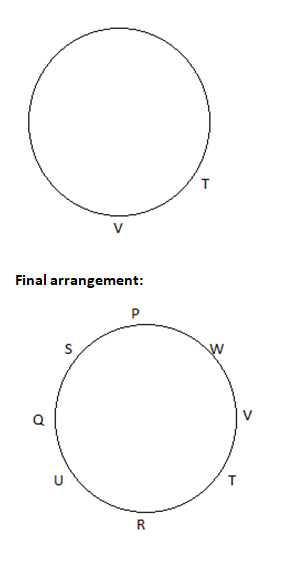### Logical Reasoning Test: Logical Reasoning test you should solve for Aptitude Preparation

Logical reasoning tests are based on a combination of skills: your ability to analyse the given problem set, figure out the important pieces of information, and use that information logically to arrive at the answer. The most important aspect here is presence of mind and exposure to different types of problems. Once you practice a number of logical reasoning tests, you are in a much better position to solve these questions. All these logical reasoning tests are essentially based on patterns which repeat. Once you get used to them, your performance improves.

Directions for the question set:
P, Q, R, S, T, U, V & W are eight friends sitting in a circle facing towards the centre.
(i) W is to the immediate left of P but is not the neighbour of T and S.
(ii) U is to the immediate right of Q and V is a neighbour of T.
(iii) R is between T and U.

Question 1: What is the position of V?
(a) To the immediate right of W
(b) Between T and R
(c) Third to the right of U
(d) Second to the left of S
(e) None of these

Question 2: What is the position of S?
(a) Between Q and U
(b) To the immediate left of P
(c) Second to the right of U
(d) To the immediate left of Q
(e) None of these

Question 3: Which of the following statements is true?
(a) U is the neighbour of V
(b) V is between W and T
(c) W is between P and S
(d) T is between U and Q
(e) None of these

### Answers and Explanations: Click the down arrow to expand

Common Solution for the set:Answer 1: (c) The position of V is third to the right of U. Correct option is (c).

Answer 2: (d) Solution: The correct position of S is to the immediate left of Q. Correct option is (d)

Answer 3: (b) Solution : Hence, V is between W and T.

Extra tips for Logical Reasoning Test:
• Make sure while solving logical reasoning test, you use the diagrammatic approach for these question sets.
• By converting the information into something visual, it makes your life easier and helps you easily identify the answers.
• For questions such as the above, which is basically based on seating arrangement/circular arrangement, you need to understand how statements are written in these questions and convert these into easy to understand diagrams.